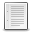File : Details

# polygonmetrics(), polyarea

Computes the length, area, CoM, and unit vector of a plane or 3D simple polygon
Details
Version
1.0
Author
Samuel Gougeon
Maintainer
Samuel Gougeon
Category
Supported Scilab Version
5.5
Creation Date
August 19, 2015Description
```            OUTPUT EXAMPLE
--------------
--> // Equilateral triangle in a cube
--> x = [0 0 1 0]; y = [0 1 1 0]; z = [0 1 0 0];
--> p = polygonmetrics([x ; y ; z])
p  =
nbvert: 3
length: 4.2426407
area: 0.8660254
com: [0.3333333,0.6666667,0.3333333]
dir: [-0.5773503,0.5773503,-0.5773503]

-----------------------------------------------------------

--> polygonmetrics

Computes the length, area, Center of Mass, and unit vector of a plane or 3D
simple polygon

SYNTAX
------
polygonmetrics     // Displays this help
pm = polygonmetrics(XY)
pm = polygonmetrics(XYZ)

PARAMETERS
----------
XY : Matrix of size (2,N) or (N,2) with N>2:
Cartesian coordinates of linked vertices of a simple plane polygon.
XYZ: Matrix of size (3,N) or (N,3) with N>2
Cartesian coordinates of linked vertices of a simple polygon.
NOTES:
* If the last point is not equal to the first one, polygonmetrics()
closes the polygon.
* The polygon must be simple, without self-intersections.
In case of a self-crossing polygon, the area value is unreliable.
* Concave as well as convex polygons are accepted.
* In 3D, the 'pseudo-polygon' is not necessarily plane.

pm : Geometrical parameters of the polygon. A structure with the following
attributes is returned:
pm.nbvert  : Number of vertices
pm.length  : Perimeter
pm.area    : Positive area
pm.com     : 3D (x,y,z) coordinates of the Center of Mass
pm.dir     : Resulting unit vector (a,b,c) orthogonal to the polygon

DESCRIPTION
-----------
The same algorithm is used in 2D and in 3D, with plane and non-plane polygons:
- The position of the Center of Mass CoM of the polygon is computed.
In 2D, it is set to the vertices centroid.
In 3D, its coordinates are the average of coordinates of its vertices.
- The vectorial area of each triangle going from the CoM to an edge is
computed. Its norm is the triangle's area. Its direction is orthogonal
to the triangle. Its sign follows the corkscrew right hand rule.
- The total oriented area is the sum of vectorial areas for all triangles
set on all edges.
- The polygon's area is the norm of the total oriented area.
- The polygon direction is the unit vector of the total oriented area.

In 2D, the resulting area matches with results from the Surveyor's formula:
area = |sum_(i=1=>N){ x(i+1)*y(i) - x(i)*y(i+1) }| / 2
The algorithm somewhat generalizes the Surveyor's formula in 3D.

--------
mesharea : http://fileexchange.scilab.org/toolboxes/370000

REFERENCE
---------
Comments, scoring and bug reports are welcome on
http://fileexchange.scilab.org/toolboxes/445000

EXAMPLES
--------
da = gda(); da.isoview = "on"; da.axes_visible = "on"; clf
yellow = color("yellow");

// Rectangle dx=2, dY = 2
x = [0 2 2 0 0]; y = [0 0 1 1 0];
subplot(4,2,1); xfpoly(x, y, yellow)
p = polygonmetrics([x ; y])

// Self-intersecting rectangle
x = [0 2 2 0 0]; y = [0 1 0 1 0];
subplot(4,2,3); xfpoly(x, y, yellow)
p = polygonmetrics([x ; y])

// Plane concave polygon:
x=[0 3 3 2 2 3 3 0 0 0.5 0.5 1 1 0 0]; y=[0 0 1 1 2 2 3 3 1.5 1.5 2 2 1 1 0];
subplot(2,2,2); xfpoly(x, y, yellow)
p = polygonmetrics([x ; y])

// Other plane polygon
r = grand(1,13,"unf",1,5);
t = linspace(0, 2*%pi, 13);
x = r.*cos(t); y = r.*sin(t);
subplot(2,2,3); xfpoly(x, y, yellow)
p = polygonmetrics([x ; y ])

// Equilateral triangle in a cube
x = [0 0 1 0]; y = [0 1 1 0]; z = [0 1 0 0];
subplot(2,2,4); param3d(x, y, z)
e = gce(); e.fill_mode = "on"; e.background = yellow;
ax = gca(); ax.isoview = "on"; ax.rotation_angles = [60 -50];
p = polygonmetrics([x ; y ; z])

da.isoview = "off"; da.axes_visible="off";
```Files (2)Screenshot
```Screenshot from polygonmetrics() examples, for thumbnail
```Miscellaneous file
```File defining the function polygonmetrics(), help contents and examples

* MAKE polygonmetrics() AVAILABLE in EVERY SESSION: Click here to see HOW TO

* Get help: Enter "polygonmetrics" without parameters
```News (0)Comments (0)